# Data

We will use winter wheat yield from the SDSU CPT trials, Brookings, SD, 2004.

arm.dat <- data.frame(
treatment=as.factor(c(1, 1, 1, 1, 2, 2, 2, 2, 3, 3, 3, 3, 4, 4, 4, 4, 5, 5, 5, 5, 6, 6, 6, 6, 7, 7, 7, 7, 8, 8, 8, 8, 9, 9, 9, 9, 10, 10, 10, 10, 11, 11, 11, 11, 12, 12, 12, 12, 13, 13, 13, 13, 14, 14, 14, 14, 15, 15, 15, 15, 16, 16, 16, 16, 17, 17, 17, 17, 18, 18, 18, 18, 19, 19, 19, 19, 20, 20, 20, 20, 21, 21, 21, 21, 22, 22, 22, 22, 23, 23, 23, 23, 24, 24, 24, 24, 25, 25, 25, 25, 26, 26, 26, 26, 27, 27, 27, 27, 28, 28, 28, 28, 29, 29, 29, 29, 30, 30, 30, 30)),
replicate=as.factor(c(1, 2, 3, 4, 1, 2, 3, 4, 1, 2, 3, 4, 1, 2, 3, 4, 1, 2, 3, 4, 1, 2, 3, 4, 1, 2, 3, 4, 1, 2, 3, 4, 1, 2, 3, 4, 1, 2, 3, 4, 1, 2, 3, 4, 1, 2, 3, 4, 1, 2, 3, 4, 1, 2, 3, 4, 1, 2, 3, 4, 1, 2, 3, 4, 1, 2, 3, 4, 1, 2, 3, 4, 1, 2, 3, 4, 1, 2, 3, 4, 1, 2, 3, 4, 1, 2, 3, 4, 1, 2, 3, 4, 1, 2, 3, 4, 1, 2, 3, 4, 1, 2, 3, 4, 1, 2, 3, 4, 1, 2, 3, 4, 1, 2, 3, 4, 1, 2, 3, 4)),
assessment1=c(75.617653, 80.66712, 82.843376, 93.57714, 84.40242, 86.52787, 61.671814, 82.287865, 113.29344, 91.63213, 48.647705, 74.430496, 99.82525, 66.746964, 86.28244, 92.60787, 113.59026, 96.95918, 110.62933, 104.42089, 110.47004, 81.687996, 99.33854, 93.43282, 110.61312, 81.10134, 102.89175, 104.79193, 111.46162, 94.32498, 80.945824, 113.32862, 95.15166,
72.513535, 71.86743, 80.078705, 110.08195, 69.24999, 91.50011, 94.22576, 110.53378, 51.12890, 103.00581, 105.96031, 107.37270, 86.92945, 90.28297, 101.01584, 97.28788, 74.78449, 91.87453, 88.04466, 100.38980, 77.724686, 90.82440, 97.06618, 115.39878, 98.25053, 103.10676, 96.43465, 111.09910, 101.90965, 80.26172, 99.38879, 100.57923, 73.94340, 92.05831,
94.54791, 109.94821, 80.36036, 98.51617, 105.34106, 103.02499, 80.31730, 85.19707, 97.42909, 110.71747, 61.84912, 88.59760, 98.67880, 106.45267, 78.201775, 51.05330, 101.50252, 114.33939, 91.05904, 101.76766, 103.30523, 104.01662, 71.34833, 81.88724, 100.99155, 102.55299, 80.345673, 52.576954, 100.23418, 103.55698, 43.580173, 77.85446, 101.67175, 88.68204,
86.46480, 84.26635, 85.31037, 105.37363, 75.68684, 81.17570, 81.61174, 95.48573, 54.13223, 101.19824, 100.14119, 104.45412, 99.29804, 94.36803, 96.90421, 69.557526, 81.38324, 71.06462, 88.56049)
)

## Introduction

Many statistical models make the assumption that errors are normally distributed. Statistical moments are as set of calculations commonly used to test this assumption.

## First moment

Assume we have a series of obervations $$X = x_1, x_2, \dots x_n$$. We denote the first (raw) moment as

$E[X] = \mu$ Further moments of order $$k$$ can then be defined as $$E[(X-\mu)^k]$$. Thus, the second raw moment is

$E[(X-\mu)^2] = \mu_2 = \sigma ^2$

Further moments can be normalized by

$\frac{\mu_k}{\sigma ^k} = \frac{E[(X-\mu)^k]} {\sigma ^k}$

The third moment is commonly referred to as skewness, while the fourth moment is kurtosis.

## Standard Deviation

We commonly estimate standard deviation ($$\sigma ^2$$) using

$s^2 = \frac{\sum_i (x_i - \overline{x})^2}{N-1}$

x <- arm.dat$assessment1 N <- length(x) bar_x <- mean(x) SS <- sum((x- bar_x)^2) print(s_reml <- sqrt(SS/(N-1))) ##  15.6648 sd(x) ##  15.6648 providing restricted maximum likelihood estimate. However, when calculating skewness, it is common to calculate a maximum likelihood estimate (https://www.itl.nist.gov/div898/handbook/eda/section3/eda35b.htm) by $s^2 = \frac{\sum_i (x_i - \overline{x})^2}{N}$ print(s_ml <- sqrt(SS/N)) ##  15.59939 # Skewness Skewness can be taken as an indication that the data lack symmetry. Types of skewness - Skewness as as symptom of a non-Guassian error distribution - Skewness as a symptom of outliers A $$t$$ statistic for skewness is given by $\frac{\mu_3}{s^2/n}$ where $$s^2/n$$ is the standard error of skewness. ## Calculating Measures of Skewness ### Fisher-Pearson coefficient of skewness A calculation of skewness that follows from the formula for $$s$$ is written as $g_1 = \frac{\sum_i (x_i - \overline{x})^3}{N} \frac{1}{s_{ML}^3}$ that is, the mean of the sums of cubes, normalized by the cube of standard deviation. Note that this formula uses the ML estimate for standard deviation (https://www.itl.nist.gov/div898/handbook/eda/section3/eda35b.htm). print(g_1 <- (sum((x - bar_x)^3)/N)/(s_ml^3)) ##  -0.8056392 ### Adjusted coefficient of skewness A measure of skewness adjusted for sample size is given by (http://en.wikipedia.org/wiki/Skewness) $G_1 = \frac{\sqrt{N(N-1)} }{N-2} g_1$ This correction approaches 1 as $$N$$ becomes larger and increases $$g_1$$ by $$~5\%$$ when there are 30 samples. Ns <- 10:100 adj <- sqrt(Ns*(Ns-1))/(Ns-2) plot(Ns,adj)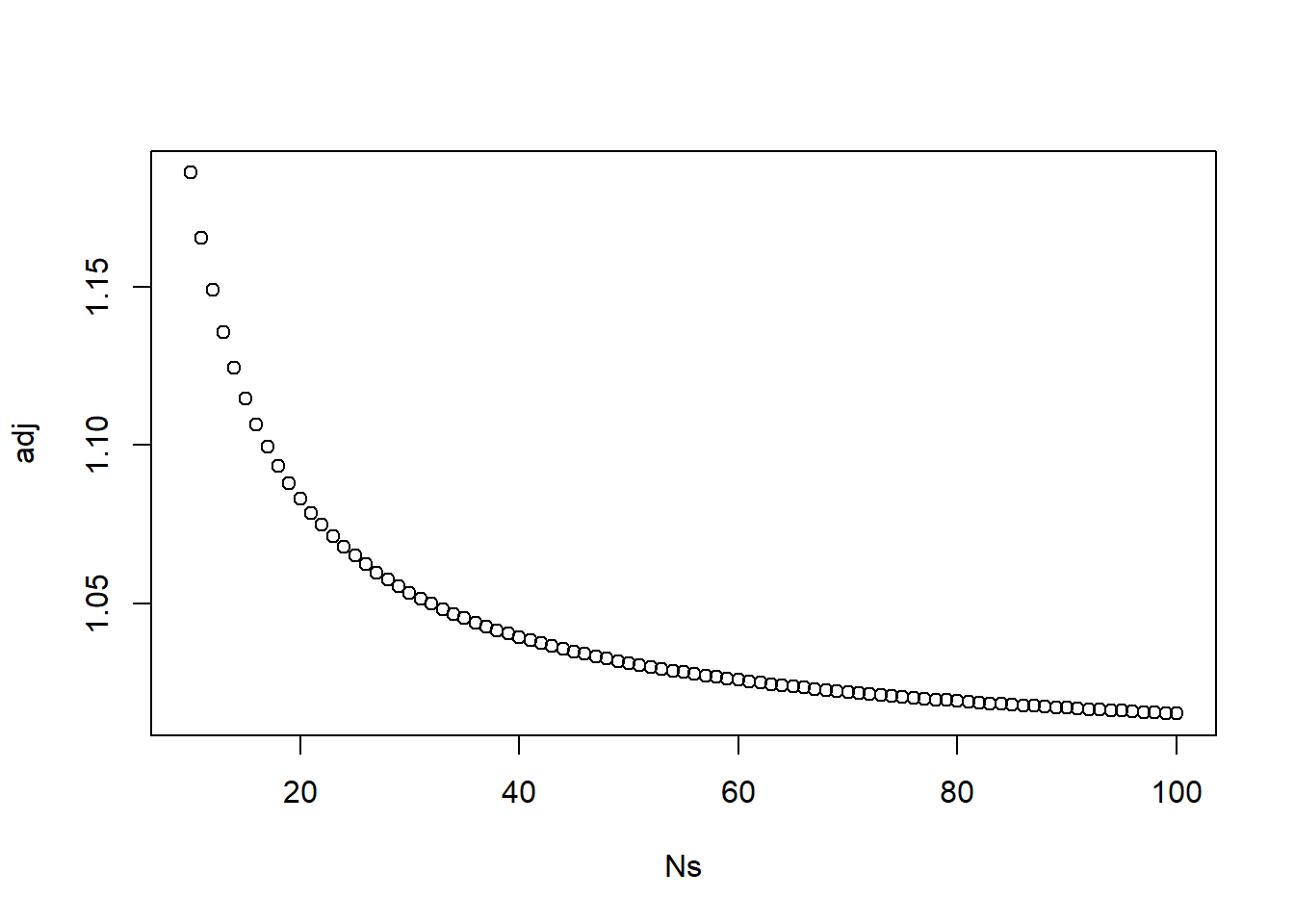print(G_1 <- g_1*sqrt(N*(N-1))/(N-2)) ##  -0.8158733 ARM has, in the past, used a sample-corrected skewness as given in http://www.anthony-vba.kefra.com/vba/excelvba-simulation-102.htm $\sum \left(\frac{X - \overline{X}}{\sigma}\right)^3 \times \frac{N}{(N-1)(N-2)}$ Function Skew(arr() As Single) Dim i As Long, n As Long Dim avg As Single, sd As Single, SumTo3 As Single n = UBound(arr) avg = Mean(arr) sd = (Var(arr)) ^ 0.5 SumTo3 = 0 For i = 1 To n SumTo3 = SumTo3 + ((arr(i) - avg) / sd) ^ 3 Next i Skew = SumTo3 * (n / ((n - 1) * (n - 2))) End Function (sum(((x - bar_x)/s_reml)^3))*(N/((N-1)*(N-2))) ##  -0.8158733 This duplicates the example A <- c(24,98,46,73,16,94,45,25,75,58) print(bar.A <- mean(A)) ##  55.4 As <- length(A) print(s.a <- sqrt(sum((A-bar.A)^2)/(As-1))) ##  29.18219 (sum(((A - bar.A)/s.a)^3))*(As/((As-1)*(As-2))) ##  0.1433405 # Kurtosis ## Calculating Kurtosis Similarly, we calculate kurtosis as $g_2 = \frac{\sum_i (x_i - \overline{x})^4}{N} \frac{1}{s_{ML}^4}$ Since the expected kurtosis of a standard normal poopulation is 3,excess kurtosis is usually reported as $g_2 = \frac{\sum_i (x_i - \overline{x})^4}{N} \frac{1}{s_{ML}^4} - 3$ print(g_2 <- (sum((x - bar_x)^4)/N)/(s_ml^4)-3) ##  0.3529375 A sample-adjusted measure of kurtosis is given by (http://en.wikipedia.org/wiki/Kurtosis) $G_2 = \frac{\sum_i (x_i - \overline{x})^4}{N} \frac{1}{s_{REML}^4} \times \frac{N(N-1)}{(N-1)(N-2)(N-3)} - 3 \frac{{(N-1)^2}}{(N-2)(N-3)}$ Note that this is written in terms of the REML estimate of standard deviation. G_2 <- (sum((x - bar_x)^4))/(s_reml^4) * (N*(N+1))/((N-1)*(N-2)*(N-3)) print(G_2 <- G_2 - (3*(N-1)^2)/((N-2)*(N-3))) ##  0.4198136 ## ARM Moments function moments <- function(x, df=0, alpha=0.05) { missing <- sum(is.na(x)) x <- x[!is.na(x)] bar_x <- mean(x) N <- length(x) if(df==0) { df <- N-1 } SS <- sum((x- bar_x)^2) s_reml <- sqrt(SS/(N-1)) s_ml <- sqrt(SS/N) g_1 <- (sum((x - bar_x)^3)/N)/(s_ml^3) G_1 <- g_1*sqrt(N*(N-1))/(N-2) SES <- sqrt((6*N*N-1)/((N-2)*(N+1)*(N+1))) skew.t <- G_1/SES skew.p <- 2*(1-pt(abs(skew.t),N-1)) g_2 <- (sum((x - bar_x)^4)/N)/(s_ml^4)-3 G_2 <- (sum((x - bar_x)^4))/(s_reml^4) * (N*(N+1))/((N-1)*(N-2)*(N-3)) G_2 <- G_2 - (3*(N-1)^2)/((N-2)*(N-3)) SEK <- 2*SES*(sqrt((N^2-1)/((N-3)*(N+5)))) kurt.t <- G_2/SEK kurt.p <- 2*(1-pt(abs(kurt.t),N-1)) return(list(Mean=bar_x, Missing = missing, N = N, ErrorDF = df, SD = sqrt(SS/df), SD_reml = s_reml, SD_ml = s_ml, g_1 = g_1, G_1 = G_1, SkewnessT = skew.t, Skewness = ifelse(skew.p<1e-4,0,skew.p), g_2 = g_2, G_2 = G_2, KurtosisT = kurt.t, Kurtosis = ifelse(kurt.p<1e-4,0,kurt.p))) } moments(x) ##$Mean
##  90.75266
##
## $Missing ##  0 ## ##$N
##  120
##
## $ErrorDF ##  119 ## ##$SD
##  15.6648
##
## $SD_reml ##  15.6648 ## ##$SD_ml
##  15.59939
##
## $g_1 ##  -0.8056392 ## ##$G_1
##  -0.8158733
##
## $SkewnessT ##  -3.648335 ## ##$Skewness
##  0.0003930902
##
## $g_2 ##  0.3529375 ## ##$G_2
##  0.4198136
##
## $KurtosisT ##  0.9459764 ## ##$Kurtosis
##  0.3460775

## Skewness and Kurtosis in R

Several R libraries provide functions to calculate skewness and kurtosis.

library(agricolae)
## Warning: package 'agricolae' was built under R version 3.6.1
skewness(x)
##  -0.8158733
kurtosis(x)
##  0.4198136
library(moments)
##
## Attaching package: 'moments'
## The following objects are masked from 'package:agricolae':
##
##     kurtosis, skewness
skewness(x)
##  -0.8056392
agostino.test(x)
##
##  D'Agostino skewness test
##
## data:  x
## skew = -0.80564, z = -3.41358, p-value = 0.0006412
## alternative hypothesis: data have a skewness
kurtosis(x)
##  3.352937
anscombe.test(x)
##
##  Anscombe-Glynn kurtosis test
##
## data:  x
## kurt = 3.3529, z = 1.0348, p-value = 0.3007
## alternative hypothesis: kurtosis is not equal to 3
geary(x)
##  0.8084177
bonett.test(x)
##
##  Bonett-Seier test for Geary kurtosis
##
## data:  x
## tau = 12.61082, z = -0.54144, p-value = 0.5882
## alternative hypothesis: kurtosis is not equal to sqrt(2/pi)
library(e1071)
## Warning: package 'e1071' was built under R version 3.6.1
##
## Attaching package: 'e1071'
## The following objects are masked from 'package:moments':
##
##     kurtosis, moment, skewness
## The following objects are masked from 'package:agricolae':
##
##     kurtosis, skewness
skewness(x,type=1)
##  -0.8056392
skewness(x,type=2)
##  -0.8158733
skewness(x,type=3)
##  -0.7955897
kurtosis(x,type=1)
##  0.3529375
kurtosis(x,type=2)
##  0.4198136
kurtosis(x,type=3)
##  0.297288

To compare with ARM reported values, add the following fragments to the RMarkdown file exported from ARM.

library(moments)
skewness(Residuals$IID) agostino.test(Residuals$IID)
kurtosis(Residuals$IID)-3 anscombe.test(Residuals$IID)
library(e1071)
skewness(Residuals$IID,type=2) kurtosis(Residuals$IID,type=2)

# Examples of skewness and kurtosis

samples <- rnorm(500,10,1)
hist(samples)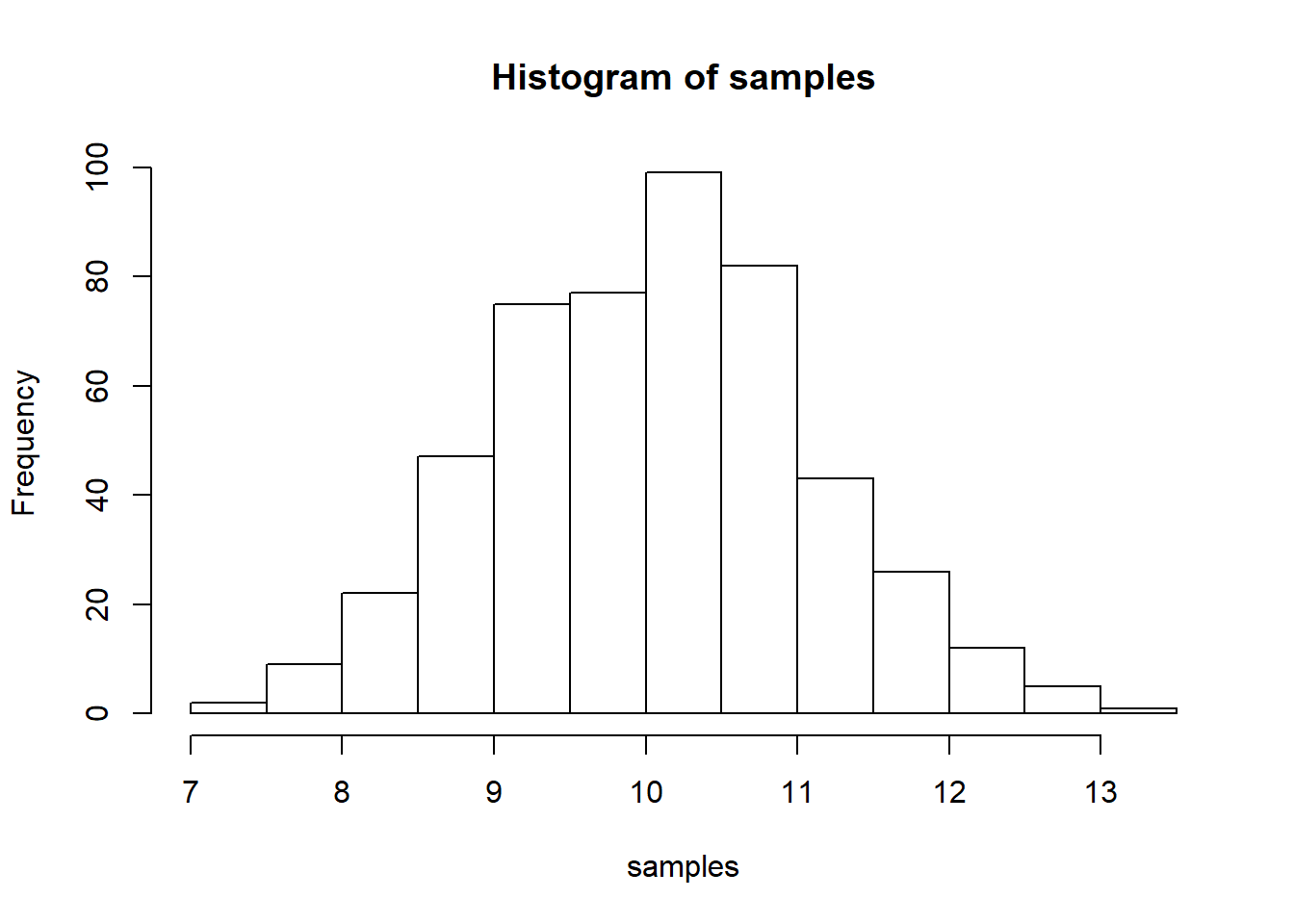qqnorm(samples)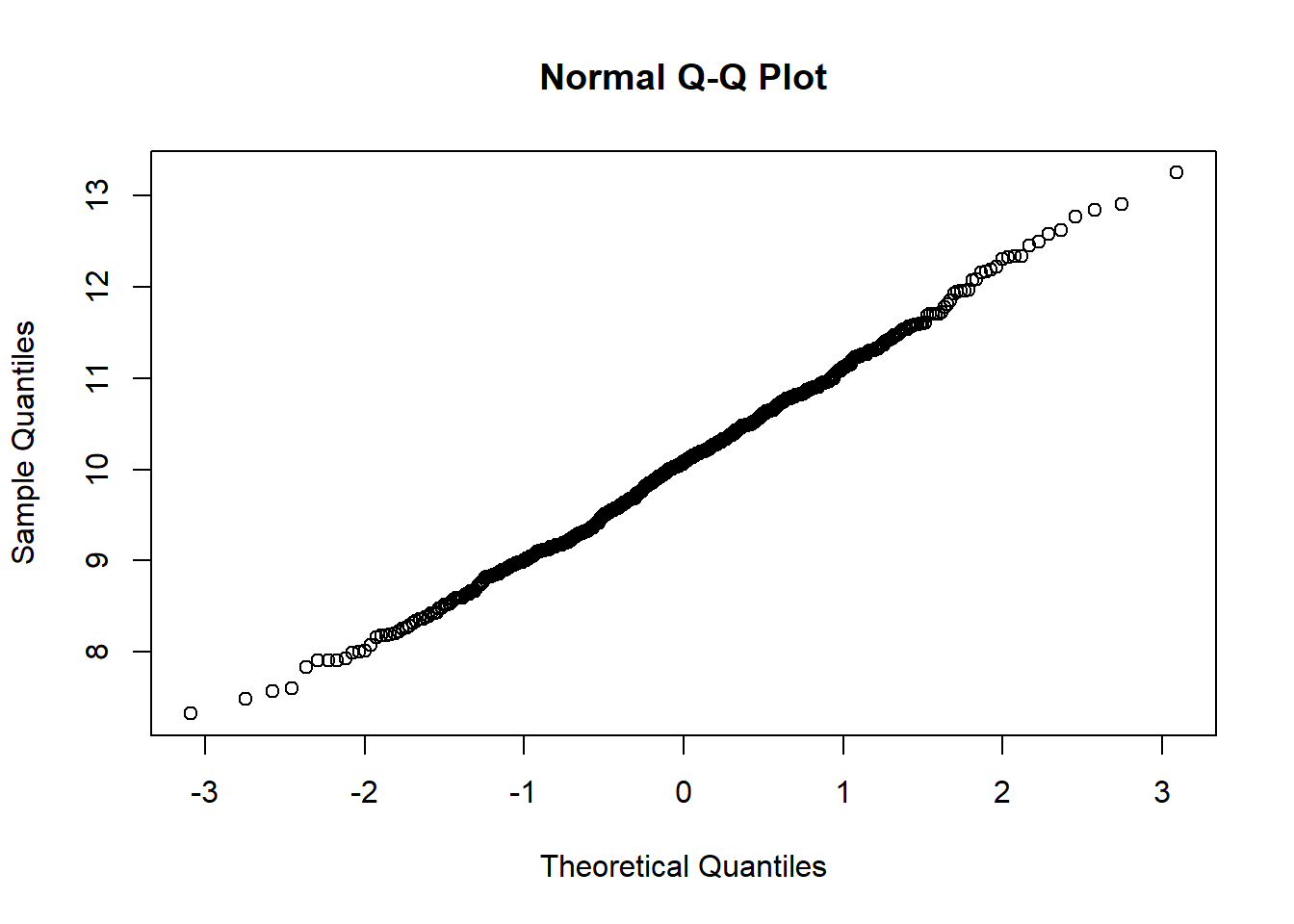moments(samples)
## $Mean ##  10.06429 ## ##$Missing
##  0
##
## $N ##  500 ## ##$ErrorDF
##  499
##
## $SD ##  1.050542 ## ##$SD_reml
##  1.050542
##
## $SD_ml ##  1.049491 ## ##$g_1
##  0.1225212
##
## $G_1 ##  0.1228902 ## ##$SkewnessT
##  1.121823
##
## $Skewness ##  0.2624774 ## ##$g_2
##  -0.2121686
##
## $G_2 ##  -0.202209 ## ##$KurtosisT
##  -0.9247668
##
## $Kurtosis ##  0.3555342 skew.inverse <- 1/(samples) hist(skew.inverse)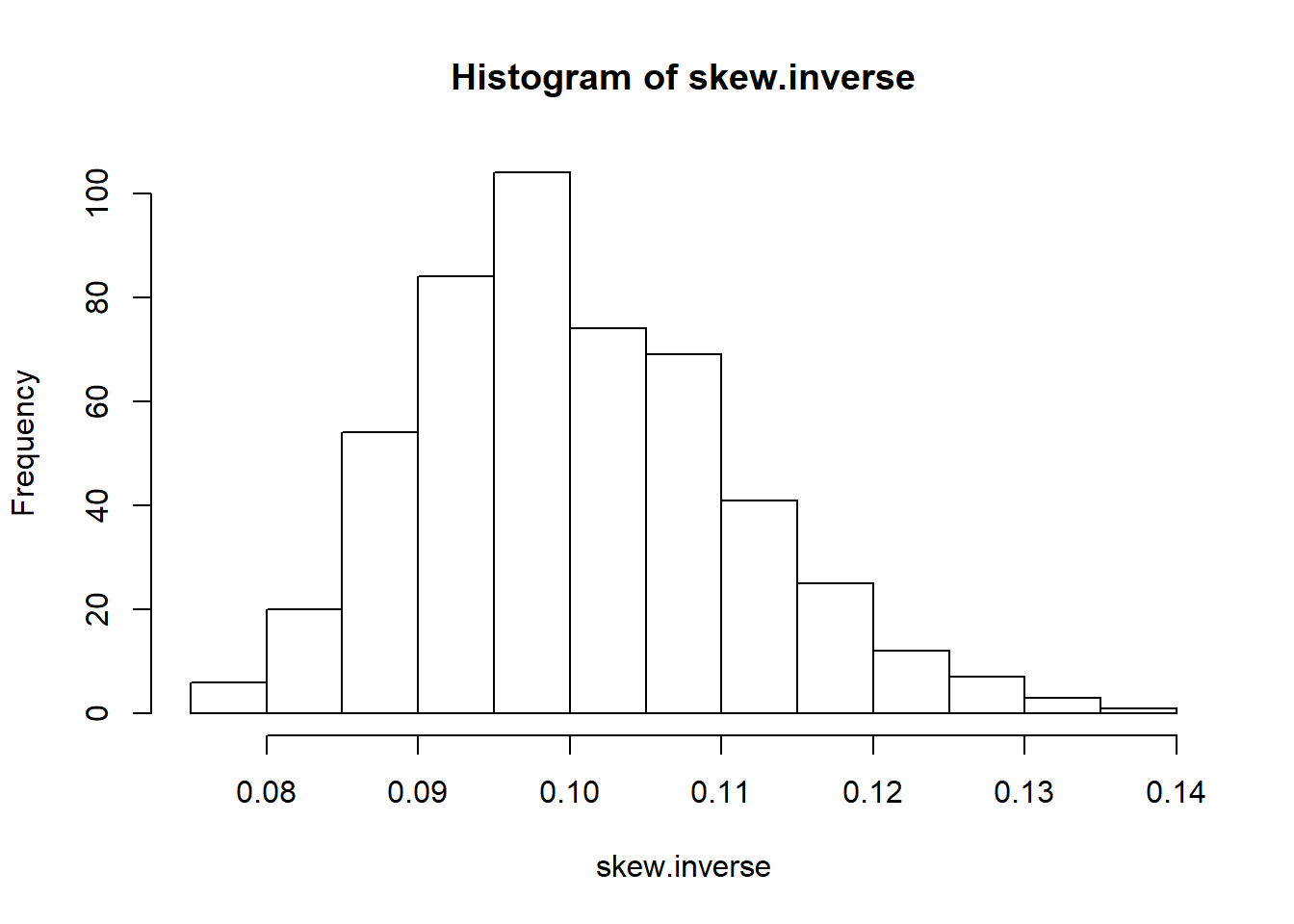qqnorm(skew.inverse)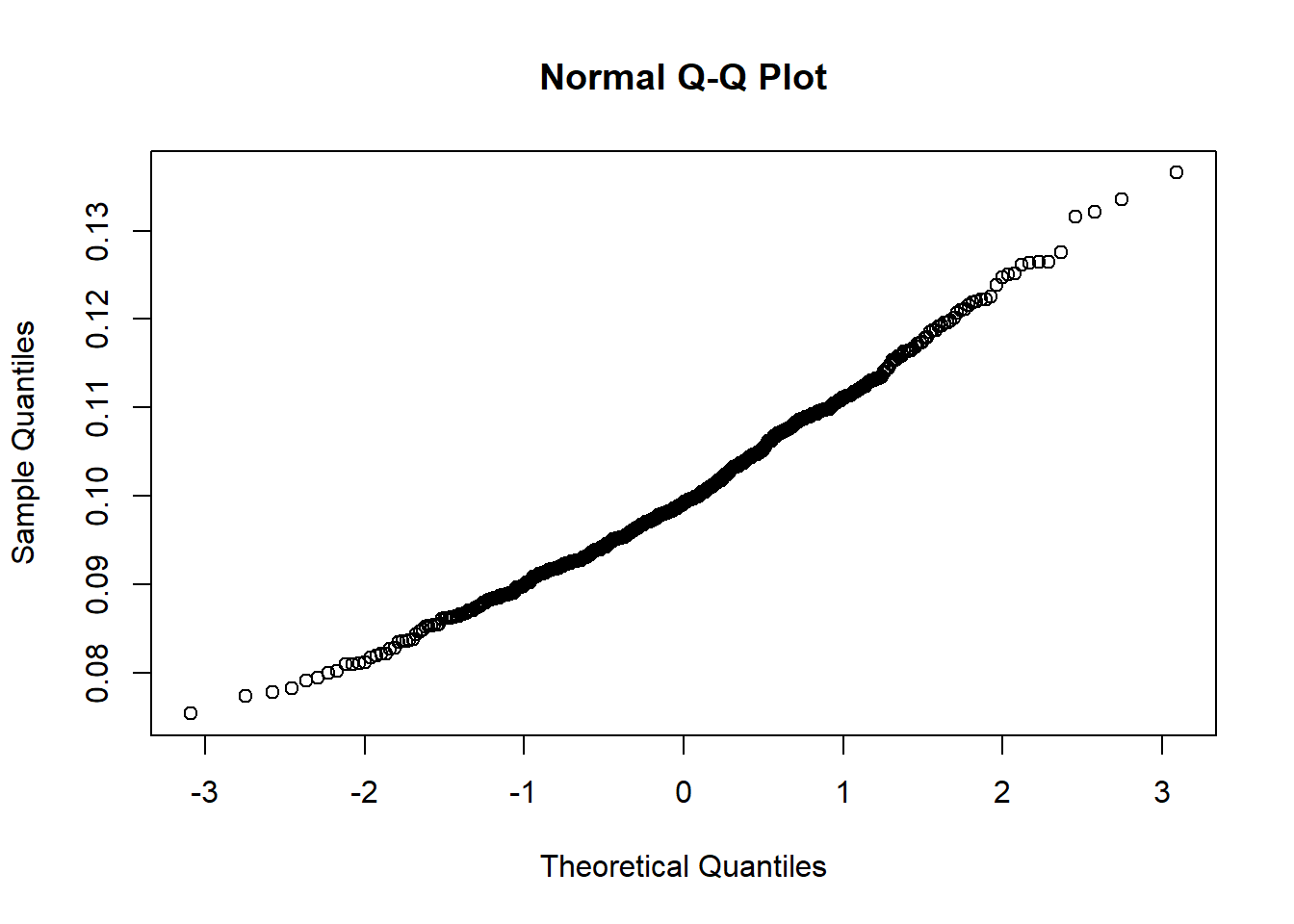moments(skew.inverse) ##$Mean
##  0.1004606
##
## $Missing ##  0 ## ##$N
##  500
##
## $ErrorDF ##  499 ## ##$SD
##  0.01065651
##
## $SD_reml ##  0.01065651 ## ##$SD_ml
##  0.01064585
##
## $g_1 ##  0.4332687 ## ##$G_1
##  0.4345735
##
## $SkewnessT ##  3.967073 ## ##$Skewness
##  0
##
## $g_2 ##  0.02118114 ## ##$G_2
##  0.03349116
##
## $KurtosisT ##  0.1531658 ## ##$Kurtosis
##  0.8783294
skew.right <- exp(samples/2)
hist(skew.right)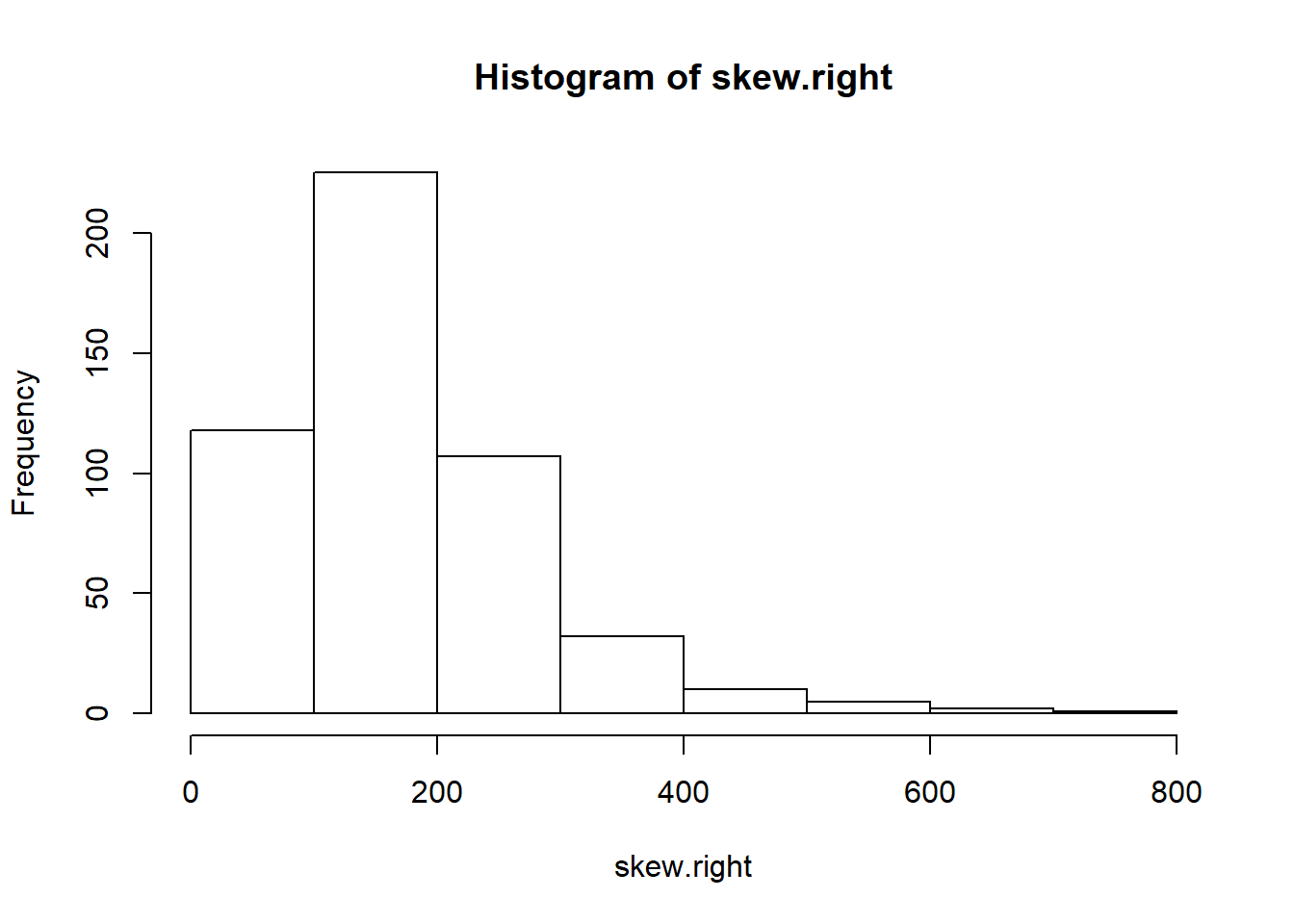qqnorm(skew.right)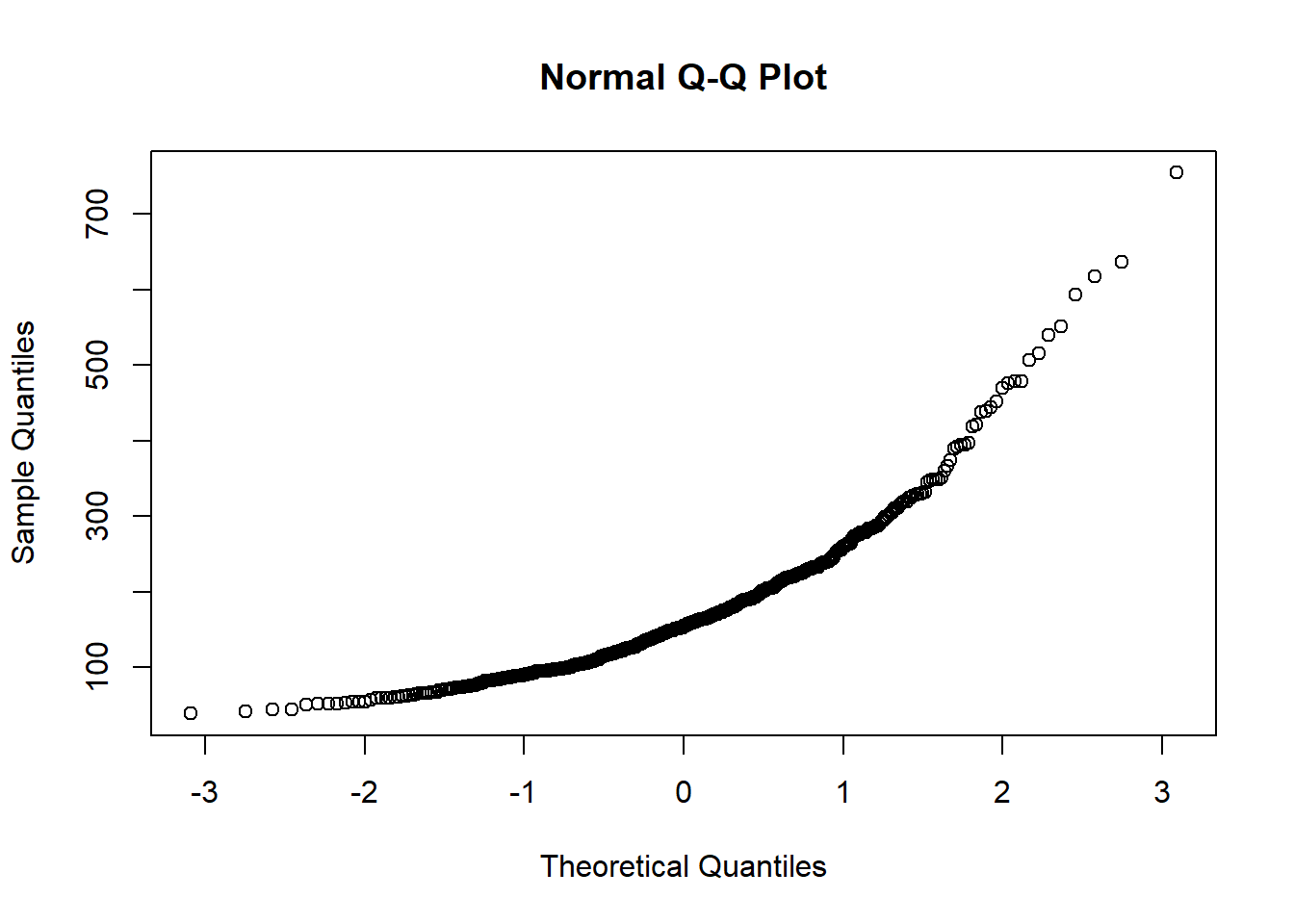moments(skew.right)
## $Mean ##  176.283 ## ##$Missing
##  0
##
## $N ##  500 ## ##$ErrorDF
##  499
##
## $SD ##  100.9267 ## ##$SD_reml
##  100.9267
##
## $SD_ml ##  100.8257 ## ##$g_1
##  1.762234
##
## $G_1 ##  1.767541 ## ##$SkewnessT
##  16.13527
##
## $Skewness ##  0 ## ##$g_2
##  4.60816
##
## $G_2 ##  4.666673 ## ##$KurtosisT
##  21.34219
##
## $Kurtosis ##  0 skew.left <- log(2*samples) hist(skew.left)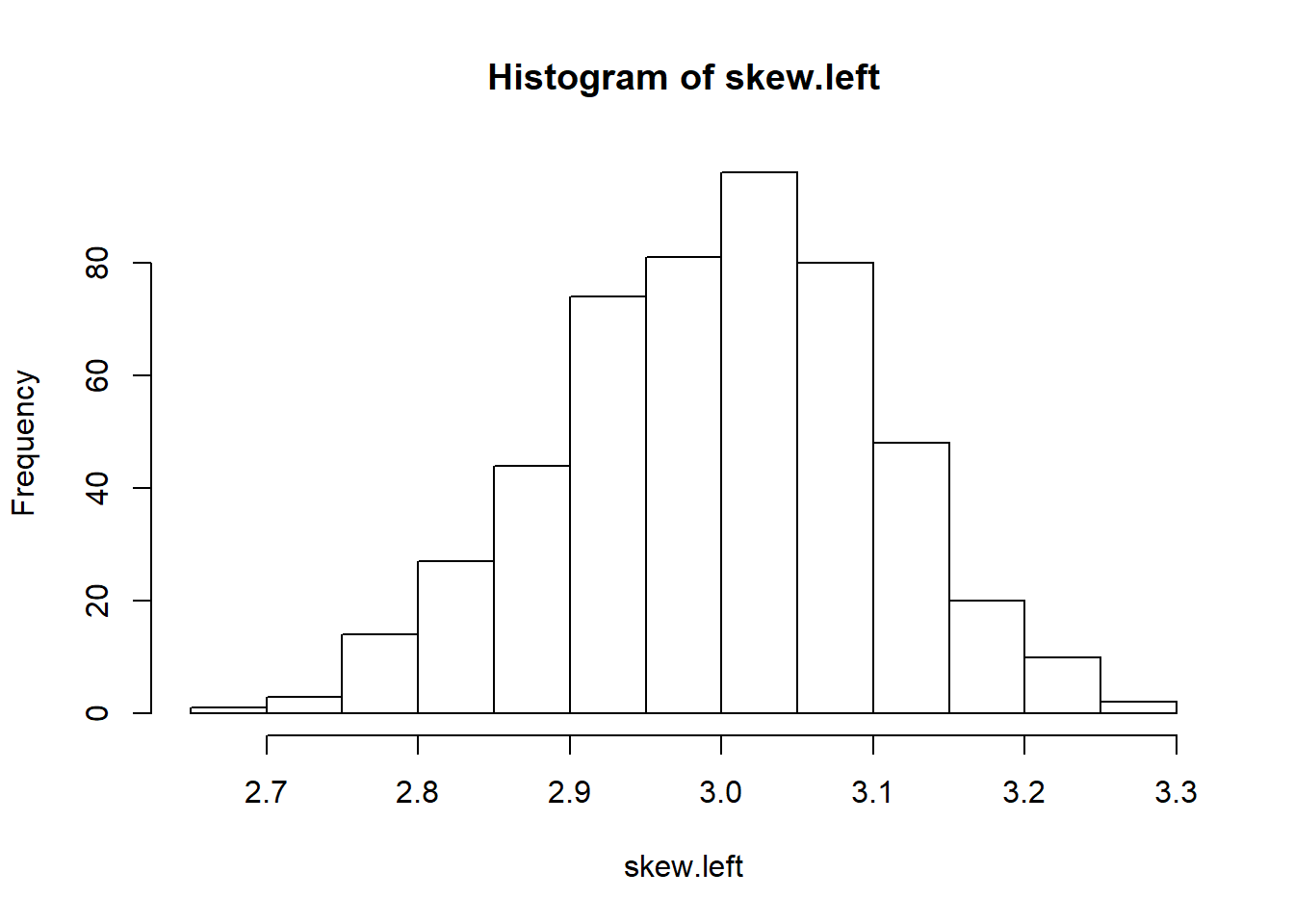qqnorm(skew.left)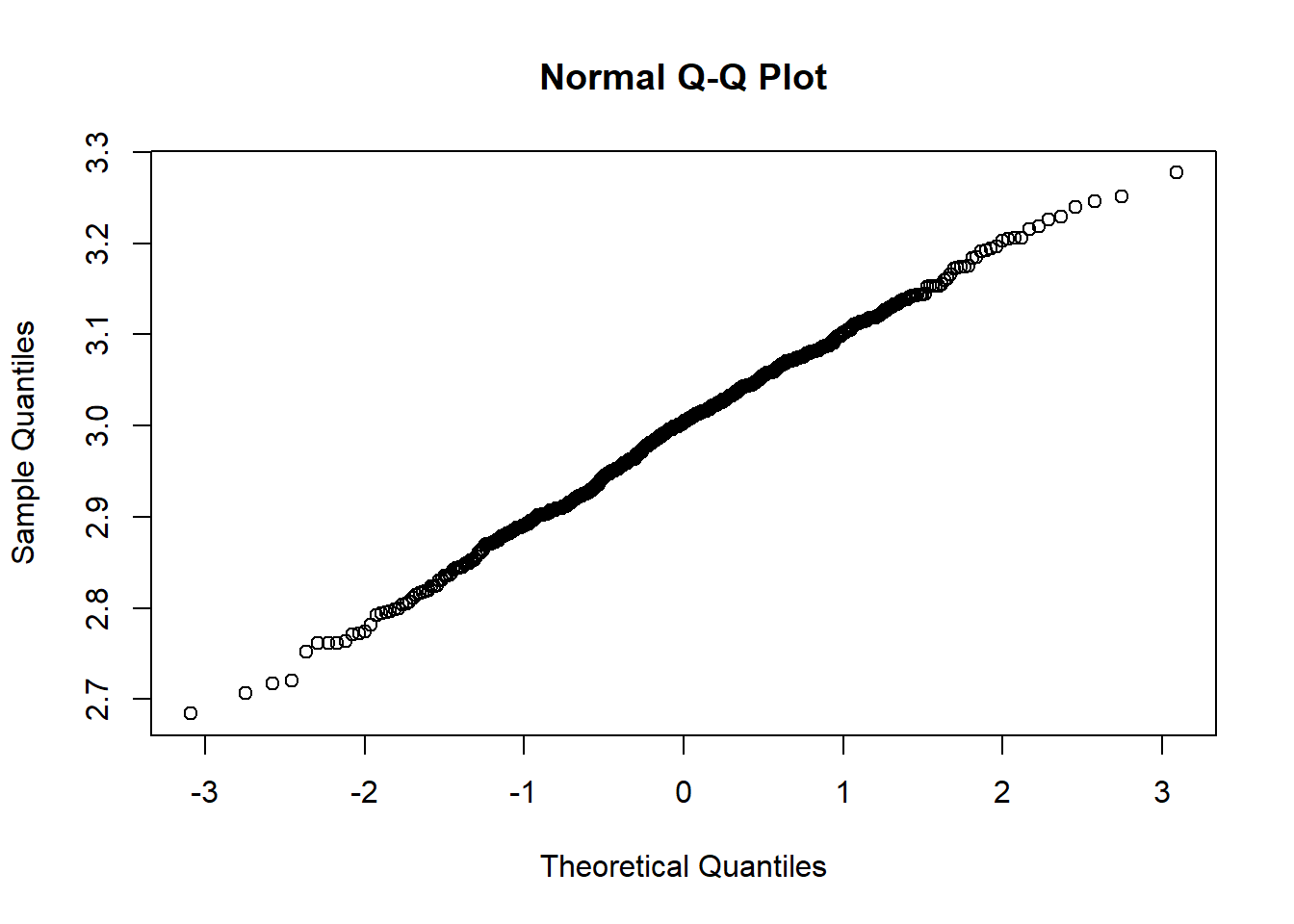moments(skew.left) ##$Mean
##  2.996668
##
## $Missing ##  0 ## ##$N
##  500
##
## $ErrorDF ##  499 ## ##$SD
##  0.1050172
##
## $SD_reml ##  0.1050172 ## ##$SD_ml
##  0.1049121
##
## $g_1 ##  -0.1534444 ## ##$G_1
##  -0.1539065
##
## $SkewnessT ##  -1.40496 ## ##$Skewness
##  0.1606555
##
## $g_2 ##  -0.2165344 ## ##$G_2
##  -0.2066187
##
## $KurtosisT ##  -0.9449337 ## ##$Kurtosis
##  0.34515